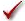## Please enable JavaScript to view this site.

 Analysis checklist: Interpolating from a standard curve

Your approach in evaluating nonlinear regression depends on your goal.

In many cases, your goal is to learn from the best-fit values. If that is your goal, view a different checklist.

If your goal is to create a standard curve from which to interpolate unknown values, your approach depends on whether this is a new or established assay.

## Established assay

If the assay is well established, then you know you are fitting the right model and know what kind of results to expect. In this case, evaluating a fit is pretty easy.Does the curve go near the points?Is the R2 'too low' compared to prior runs of this assay?

If so, look for outliers, or use Prism's automatic outlier detection.Are the confidence bands too wide?

The confidence bands let you see how accurate interpolations will be, so we suggest always plotting prediction bands when your goal is to interpolate from the curve. If your are running an established assay, you know how wide you expect the prediction bands to be.

## New assay

With a new assay, you also have to wonder about whether you picked an appropriate model.Does the curve go near the points?

Look at the graph. Does it look like the curve goes near the points.Are the confidence bands too wide?

How wide is too wide? The prediction bands show you how precise interpolations will be. Draw a horizontal line somewhere along the curve, and look at the two places where that line intercepts the confidence bands. This will be the confidence interval for the interpolation.Does the scatter of points around the best-fit curve follow a Gaussian distribution?

Least squares regression is based on the assumption that the scatter of points around the curve follows a Gaussian distribution. Prism offers three normality tests (in the Diagnostics tab) that can test this assumption (we recommend the D'Agostino test). If the P value for a normality test is low, you conclude that the scatter is not Gaussian.Could outliers be impacting your results?

Nonlinear regression is based on the assumption that the scatter of data around the ideal curve follows a Gaussian distribution. This assumption leads to the goal of minimizing the sum of squares of distances of the curve from the points. The presence of one or a few outliers (points much further from the curve than the rest) can overwhelm the least-squares calculations and lead to misleading results.

You can spot outliers by examining a graph (so long as you plot individual replicates, and not mean and error bar). But outliers can also be detected automatically. GraphPad has developed a new method for identifying outliers we call the ROUT method. You can either ask Prism to simply identify outliers or to eliminate them. These choices are adjacent on the Interpolate a Standard Curve dialog. But they are in separate tabs in the Nonlinear Regression dialog. The option to count outliers is on the Diagnostics tab, and the the option to exclude outliers is on the Fit tab.Does the curve deviate systematically from the data?

If either the runs test or the replicates test yields a low P value, then you can conclude that the curve doesn't really describe the data very well. You may have picked the wrong model.